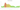L

Swazi lilangeni

how has the value of the currency changed in the last year?USD 1 = SZL 15.312

How much is10 US dollars worth inSwazi emalangeni?
At the current exchange rate, 10 US dollars is worth 153.12 Swazi emalangeni
How much is50 US dollars worth inSwazi emalangeni?
At the current exchange rate, 50 US dollars is worth 765.58 Swazi emalangeni
How much is100 US dollars worth inSwazi emalangeni?
At the current exchange rate, 100 US dollars is worth 1,531.17 Swazi emalangeni
How much is500 US dollars worth inSwazi emalangeni?
At the current exchange rate, 500 US dollars is worth 7,655.84 Swazi emalangeni
How much is2,000 US dollars worth inSwazi emalangeni?
At the current exchange rate, 2,000 US dollars is worth 30,623.36 Swazi emalangeni
How much is10 US dollars worth inSwazi emalangeni?
At the current exchange rate, 10 US dollars is worth 153.12 Swazi emalangeni
How much is50 US dollars worth inSwazi emalangeni?
At the current exchange rate, 50 US dollars is worth 765.58 Swazi emalangeni
How much is100 US dollars worth inSwazi emalangeni?
At the current exchange rate, 100 US dollars is worth 1,531.17 Swazi emalangeni
How much is500 US dollars worth inSwazi emalangeni?
At the current exchange rate, 500 US dollars is worth 7,655.84 Swazi emalangeni
How much is2,000 US dollars worth inSwazi emalangeni?
At the current exchange rate, 2,000 US dollars is worth 30,623.36 Swazi emalangeni